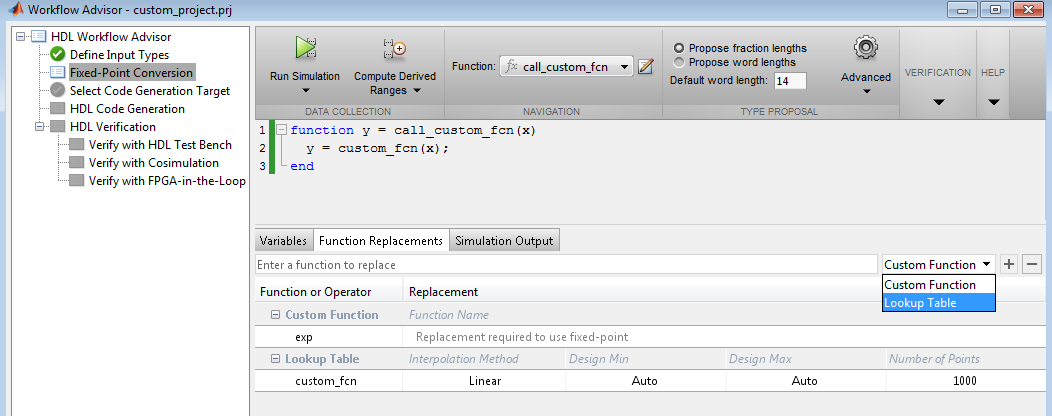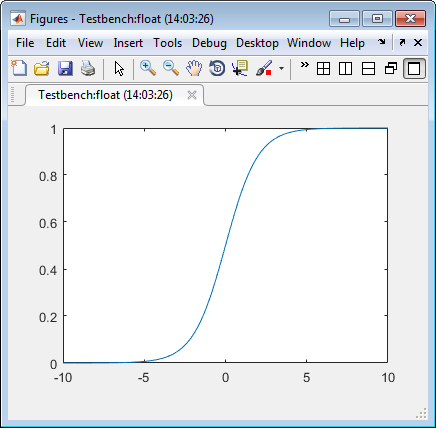## Replace a Custom Function with a Lookup Table

With HDL Coder™, you can generate lookup table approximations for functions that do not support fixed-point types, and replace your own functions. To replace a custom function with a Lookup Table, use the HDL Coder app, or the `fiaccel` `codegen` function.

### Using the HDL Coder App

This example shows how to replace a custom function with a Lookup Table using the HDL Coder app.

Create Algorithm and Test Files

In a local, writable folder:

1. Create a MATLAB® function, `custom_fcn`, which is the function that you want to replace.

```function y = custom_fcn(x) y = 1./(1+exp(-x)); end```
2. Create a wrapper function that calls `custom_fcn`.

```function y = call_custom_fcn(x) y = custom_fcn(x); end```
3. Create a test file, `custom_test`, which uses `call_custom_fcn`.

```close all x = linspace(-10,10,1e3); for itr = 1e3:-1:1 y(itr) = call_custom_fcn( x(itr) ); end plot( x, y ); ```

Create and Set up a HDL Coder Project

1. Navigate to the work folder that contains the file for this example.

2. To open the HDL Coder app, in the MATLAB command prompt, enter `hdlcoder`. Set Name to `custom_project.prj` and click . The project opens in the MATLAB workspace.

3. In the project window, on the MATLAB Function tab, click the Add MATLAB function link. Browse to the file `call_custom_fcn.m`, and then click to add the file to the project.

Define Input Types

1. To define input types for `call_custom_fcn.m`, on the MATLAB Function tab, click Autodefine types.

2. Add `custom_test` as a test file, and then click .

From the test file, HDL Coder determines that `x` is a scalar double.

3. Click .

Replace custom_fcn with Lookup Table

1. To open the HDL Workflow Advisor, click , and in the Workflow Advisor window, click Fixed-Point Conversion.

2. To replace `custom_fcn` with a Lookup Table, on the Function Replacements tab, enter `custom_fcn`, select `Lookup Table`, and then click +.

By default, the lookup table uses linear interpolation, 1000 points, and design minimum and maximum values that the app detects by running a simulation or computing derived ranges.3. Under Run Simulation, select ```Log data for histogram```, and then click Run Simulation. Verify that `custom_test` file is selected as the test file.

The simulation runs and the tool displays simulation minimum and maximum ranges on the Variables tab. HDL Coder plots the simulation results in the MATLAB Editor.Validate Fixed-Point Types

1. In the Proposed Type column, verify that the fixed-point types proposed by software cover the full simulation range. To view logged histogram data for a variable, click its Proposed Type field.

The histogram provides range information and the percentage of simulation range that the proposed data type covers.2. To validate the build by using the proposed types, click .

The software validates the proposed types and generates a fixed-point code, `call_custom_fcn_fixpt`.

3. To view the generated fixed-point code, click the `call_custom_fcn_fixpt` link.

The generated fixed-point function, `call_custom_fcn_fixpt.m`, calls this approximation instead of calling `custom_fcn`.

```function y = call_custom_fcn_fixpt(x) fm = get_fimath(); y = fi(replacement_custom_fcn(x), 0, 14, 14, fm); end function fm = get_fimath() fm = fimath('RoundingMethod', 'Floor',... 'OverflowAction', 'Wrap',... 'ProductMode','FullPrecision',... 'MaxProductWordLength', 128,... 'SumMode','FullPrecision',... 'MaxSumWordLength', 128); end```

### From the Command Line

Prerequisites

To complete this example, you must install the following products:

• MATLAB

• Fixed-Point Designer™

• C compiler

You can use `mex -setup` to change the default compiler. See Change Default Compiler.

Create a MATLAB function, `custom_fcn.m`. This is the function that you want to replace.

```function y = custom_fcn(x) y = 1./(1+exp(-x)); end```

Create a wrapper function that calls `custom_fcn.m`.

```function y = call_custom_fcn(x) y = custom_fcn(x); end```

Create a test file, `custom_test.m`, that uses `call_custom_fcn.m`.

```close all x = linspace(-10,10,1e3); for itr = 1e3:-1:1 y(itr) = call_custom_fcn( x(itr) ); end plot( x, y ); ```

Create a function replacement configuration object to approximate `custom_fcn`. Specify the function handle of the custom function and set the number of points to use in the lookup table to 50.

`q = coder.approximation('Function','custom_fcn','CandidateFunction',@custom_fcn, 'NumberOfPoints',50);`

Create a `coder.FixptConfig` object, `fixptcfg`. Specify the test file name and enable numerics testing. Associate the function replacement configuration object with the fixed-point configuration object.

```fixptcfg = coder.config('fixpt'); fixptcfg.TestBenchName = 'custom_test'; fixptcfg.TestNumerics = true; fixptcfg.addApproximation(q);```

Generate fixed-point MATLAB code.

`codegen -float2fixed fixptcfg call_custom_fcn`

`codegen` generates fixed-point MATLAB code in `call_custom_fcn_fixpt.m`.

To view the generated fixed-point code, click the link to `call_custom_fcn_fixpt`.

The generated code contains a lookup table approximation, `replacement_custom_fcn`, for the `custom_fcn` function. The fixed-point conversion process infers the ranges for the function and then uses an interpolated lookup table to replace the function. The lookup table uses 50 points as specified. By default, it uses linear interpolation and the minimum and maximum values detected by running the test file.

The generated fixed-point function, `call_custom_fcn_fixpt`, calls this approximation instead of calling `custom_fcn`.

```function y = call_custom_fcn_fixpt(x) fm = get_fimath(); y = fi(replacement_custom_fcn(x), 0, 14, 14, fm); end```

You can now test the generated fixed-point code and compare the results against the original MATLAB function. If the behavior of the generated fixed-point code does not match the behavior of the original code closely enough, modify the interpolation method or number of points used in the lookup table and then regenerate code.